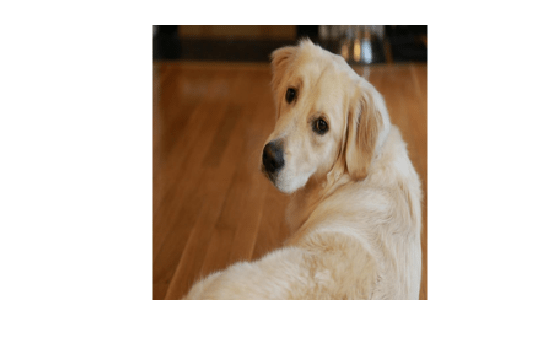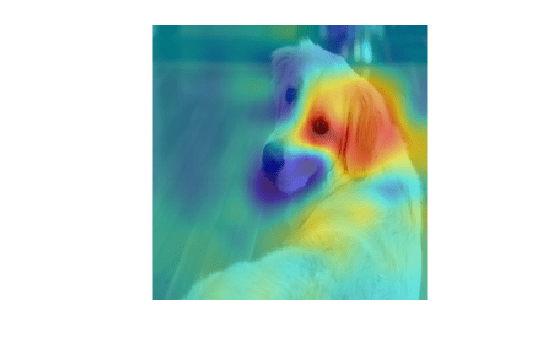# occlusionSensitivity

Determine how input data affects output activations by occluding input

## Syntax

``scoreMap = occlusionSensitivity(net,X,label)``
``activationMap = occlusionSensitivity(net,X,layer,channel)``
``___ = occlusionSensitivity(___,Name,Value)``

## Description

example

````scoreMap = occlusionSensitivity(net,X,label)` computes a map of the change in classification score for the classes specified by `label` when parts of the input data `X` are occluded with a mask. The change in classification score is relative to the original data without occlusion. The occluding mask is moved across the input data, giving a change in classification score for each mask location. Use an occlusion map to identify the parts of your input data that most impact the classification score. Areas in the map with higher positive values correspond to regions of input data that contribute positively to the specified classification label. The network must contain a `softmaxLayer` followed by a `classificationLayer`.```
````activationMap = occlusionSensitivity(net,X,layer,channel)` computes a map of the change in total activation for the specified layer and channel when parts of the input data `X` are occluded with a mask. The change in activation score is relative to the original data without occlusion. Areas in the map with higher positive values correspond to regions of input data that contribute positively to the specified channel activation, obtained by summing over all spatial dimensions for that channel. ```
````___ = occlusionSensitivity(___,Name,Value)` specifies options using one or more name-value pair arguments in addition to the input arguments in previous syntaxes. For example, `'Stride',50` sets the stride of the occluding mask to 50 pixels. ```

## Examples

collapse all

`net = googlenet;`

Import the image and resize to match the input size for the network.

```X = imread("sherlock.jpg"); inputSize = net.Layers(1).InputSize(1:2); X = imresize(X,inputSize);```

Display the image.

`imshow(X)`Classify the image to get the class label.

`label = classify(net,X)`
```label = categorical golden retriever ```

Use `occlusionSensitivity` to determine which parts of the image positively influence the classification result.

`scoreMap = occlusionSensitivity(net,X,label);`

Plot the result over the original image with transparency to see which areas of the image affect the classification score.

```figure imshow(X) hold on imagesc(scoreMap,'AlphaData',0.5); colormap jet```The red parts of the map show the areas which have a positive contribution to the specified label. The dog's left eye and ear strongly influence the network's prediction of `golden retriever`.

You can get similar results using the gradient class activation mapping (Grad-CAM) technique. Grad-CAM uses the gradient of the classification score with respect to the last convolutional layer in a network in order to understand which parts of the image are most important for classification. For an example, see Grad-CAM Reveals the Why Behind Deep Learning Decisions.

## Input Arguments

collapse all

Trained network, specified as a `SeriesNetwork` object or a `DAGNetwork` object. You can get a trained network by importing a pretrained network or by training your own network using the `trainNetwork` function. For more information about pretrained networks, see Pretrained Deep Neural Networks.

`net` must contain a single input layer. The input layer must be an `imageInputLayer`.

Observation to occlude, specified as a numeric array. You can calculate the occlusion map of one observation at a time. For example, specify a single image to understand which parts of that image affect classification results.

Data Types: `single` | `double` | `int8` | `int16` | `int32` | `int64` | `uint8` | `uint16` | `uint32` | `uint64`

Class label used to calculate change in classification score, specified as a categorical, a character array, or a string array.

If you specify `label` as a vector, the software calculates the change in classification score for each class label independently. In that case, `scoreMap(:,:,i)` corresponds to the occlusion map for the `i`th element in `label`.

Data Types: `char` | `string` | `categorical`

Layer used to calculate change in activation, specified as a character vector or a string scalar. Specify `layer` as the name of the layer in `net` for which you want to compute the change in activations.

Data Types: `char` | `string`

Channel used to calculate change in activation, specified as scalar or vector of channel indices. The possible choices for `channel` depend on the selected layer. For example, for convolutional layers, the `NumFilters` property specifies the number of output channels. You can use `analyzeNetwork` to inspect the network and find out the number of output channels for each layer.

If `channel` is specified as a vector, the change in total activation for each specified channel is calculated independently. In that case, `activationMap(:,:,i)` corresponds to the occlusion map for the `i`th element in `channel`.

The function computes the change in total activation due to occlusion. The total activation is computed by summing over all spatial dimensions of the activation of that channel. The occlusion map corresponds to the difference between the total activation of the original data with no occlusion and the total activation for the occluded data. Areas in the map with higher positive values correspond to regions of input data that contribute positively to the specified channel activation.

Data Types: `single` | `double` | `int8` | `int16` | `int32` | `int64` | `uint8` | `uint16` | `uint32` | `uint64`

### Name-Value Pair Arguments

Specify optional comma-separated pairs of `Name,Value` arguments. `Name` is the argument name and `Value` is the corresponding value. `Name` must appear inside quotes. You can specify several name and value pair arguments in any order as `Name1,Value1,...,NameN,ValueN`.

Example: `'MaskSize',75,'OutputUpsampling','nearest'` uses an occluding mask with size 75 pixels along each side, and uses nearest-neighbor interpolation to upsample the output to the same size as the input data

Size of occluding mask, specified as the comma-separated pair consisting of `'MaskSize'` and one of the following.

• `'auto'` — Use a mask size of 20% of the input size, rounded to the nearest integer.

• A vector of the form `[h w]`— Use a rectangular mask with height `h` and width `w`.

• A scalar — Use a square mask with height and width equal to the specified value.

Example: `'MaskSize',[50 60]`

Step size for traversing the mask across the input data, specified as the comma-separated pair consisting of `'Stride'` and one of the following.

• `'auto'` — Use a stride of 10% of the input size, rounded to the nearest integer.

• A vector of the form `[a b]`— Use a vertical stride of `a` and a horizontal stride of `b`.

• A scalar — Use a stride of the specified value in both the vertical and horizontal directions.

Example: `'Stride',30`

Replacement value of occluded region, specified as the comma-separated pair consisting of `'MaskValue'` and one of the following.

• `'auto'` — Replace occluded pixels with the channel-wise mean of the input data.

• A scalar — Replace occluded pixels with the specified value.

• A vector — Replace occluded pixels with the value specified for each channel. The vector must contain the same number of elements as the number of output channels of the layer.

Example: `'MaskValue',0.5`

Output upsampling method, specified as the comma-separated pair consisting of `'OutputUpsampling'` and one of the following.

• `'bicubic'` — Use bicubic interpolation to produce a smooth map the same size as the input data.

• `'nearest'` — Use nearest-neighbor interpolation expand the map to the same size as the input data. The map indicates the resolution of the occlusion computation with respect to the size of the input data.

• `'none'` — Use no upsampling. The map can be smaller than the input data.

If `'OutputUpsampling'` is `'bicubic'` or `'nearest'`, the computed map is upsampled to the size of the input data using the `imresize` function.

Example: `'OutputUpsampling','nearest'`

Edge handling of the occluding mask, specified as the comma-separated pair consisting of `'MaskClipping'` and one of the following.

• `'on'` — Place the center of the first mask at the top-left corner of the input data. Masks at the edges of the data are not full size.

• `'off'` — Place the top-left corner of the first mask at the top-left corner of the input data. Masks are always full size. If the values of the `MaskSize` and `Stride` options mean that some masks extend past the boundaries of the data, those masks are excluded.

For non-image input data, you can ensure you always occlude the same amount of input data using the option `'MaskClipping','off'`. For example, for word embeddings data, you can ensure the same number of words are occluded at each point.

Example: `'MaskClipping','off'`

Size of the mini-batch to use to compute the map of change in classification score, specified as the comma-separated pair consisting of `'MiniBatchSize'` and a positive integer.

A mini-batch is a subset of the set of occluded images as the mask is moved across the input image. All occluded images are used to calculate the map; the mini-batch determines the number of images that are passed to the network at once. Larger mini-batch sizes lead to faster computation, at the cost of more memory.

Example: `'MiniBatchSize',256`

Hardware resource for computing map, specified as the comma-separated pair consisting of `'ExecutionEnvironment'` and one of the following.

• `'auto'` — Use a GPU if one is available. Otherwise, use the CPU.

• `'cpu'` — Use the CPU.

• `'gpu'` — Use the GPU.

The GPU option requires Parallel Computing Toolbox™. To use a GPU for deep learning, you must also have a CUDA® enabled NVIDIA® GPU with compute capability 3.0 or higher. If you choose the `'ExecutionEnvironment','gpu'` option and Parallel Computing Toolbox or a suitable GPU is not available, then the software returns an error.

Example: `'ExecutionEnvironment','gpu'`

## Output Arguments

collapse all

Map of change of classification score, returned as a numeric matrix or a numeric array. The change in classification score is calculated relative to the original input data without occlusion. Areas in the map with higher positive values correspond to regions of input data that contribute positively to the specified classification label.

If `label` is specified as a vector, the change in classification score for each class label is calculated independently. In that case, `scoreMap(:,:,i)` corresponds to the occlusion map for the `i`th element in `label`.

Map of change of total activation, returned as a numeric matrix or a numeric array.

The function computes the change in total activation due to occlusion. The total activation is computed by summing over all spatial dimensions of the activation of that channel. The occlusion map corresponds to the difference between the total activation of the original data with no occlusion and the total activation for the occluded data. Areas in the map with higher positive values correspond to regions of input data that contribute positively to the specified channel activation.

If `channels` is specified as a vector, the change in total activation for each specified channel is calculated independently. In that case, `activationMap(:,:,i)` corresponds to the occlusion map for the `i`th element in `channel`.

Introduced in R2019b